IF YOU CAN SEE THIS go to /sfa/Nuprl/Shared/Xindentation_hack_doc.html

### Intensional Types

Type Expressions denote partial equivalence relations between expressions (see Types). Such a relation serves as a way of notating values constructively. This engenders a natural equivalence relation between type expressions themselves, namely extensional equality, i.e. whether they denote the same type. We sometimes express this equality as "A =ext B".

The designers of Nuprl chose to employ another partial equivalence relation between type expressions that is finer than "extensional" equality, and is therefore "intensional". This serves also to constrain construction of type expressions, since not every (extensional) type expression will be related even to itself by this relation.

When we talk about types we often mean type expressions modulo this intensional equality, that is, equivalence classes of type expressions under this intensional equality, restricted to those type expressions intensionally equal to themselves. An intensional type expression is a type expression that is intensionally equal to itself.

In particular, "x:A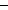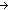B(x)" and "x:CD(x)" are stipulated to be intensionally equal just when "A" and "C" are, and further when for all expressions r and s such that r = s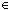A, "B(r)" and "D(s)" are intensionally equal. It is similar with most of the other type constructors; intensionally equal types have the same outermost operator, and intensionally equal corresponding components. An exception is equality between Quotient Types.

This entails that there are extensional type expressions that are not intensional type expressions. The expressions "Void" and "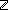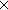Void" both denote the empty type, and so are extensionally equal, but are not intensionally equal expressions due to their differing outermost structure. Let us use this to build an extensional type expression that is not an intensional type expression.

Consider the expression "x:(mod 2)if x=0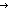Void ;Void fi".
This denotes a type extensionally since no matter what member of (mod 2) is substituted for x in "if x=0Void ;Void fi" the result is an expression for the empty type, i.e. "if x=0Void ;Void fi" denotes a(n extensional) type-valued function over (mod 2). But (0 = 2mod 2), and even though "if 0=0Void ;Void fi" and "if 2=0Void ;Void fi" denote the same (extensional) type, they denote distinct intensional types, since the first evaluates to "Void" and the second evaluates to "Void". Hence, "x:(mod 2)if x=0Void ;Void fi" is not intensionally equal to itself, and so is not an intensional type expression.

Universes are examples of types whose members are intensional types rather than (extensional) types. Here is the argument, which also shows the sense in which this is so: Void is Type{1}Void, extensionally, but Void is a member of Type{1} whereas Type{1}Void is not.

(March 2001 - sfa )